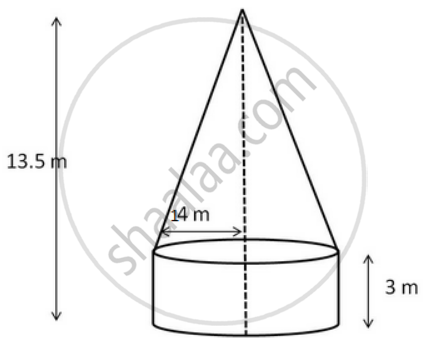Advertisement Remove all ads

# A Tent is in the Shape of a Right Circular Cylinder up to a Height of 3 M and Conical Above It. the Total Height of the Tent is 13.5 M and the Radius of Its Base is 14 M. Find the Cost of - Mathematics

Sum

A tent is in the shape of a right circular cylinder up to a height of 3 m and conical above it. The total height of the tent is 13.5 m and the radius of its base is 14 m. Find the cost of cloth required to make the tent at the rate of Rs 80 per square metre.

Advertisement Remove all ads

#### Solutionadius of the cylinder = 14 m
Radius of the base of the cone = 14 m
Height of the cylinder (h) = 3 m
Total height of the tent = 13.5 m
Surface area of the cylinder =2pirh =(2xx22/7xx14xx3)m^2 = 264  m^2

Height of the cone= Total height - Height of cone = (13.5 - 3)m =10.5 m

"surface area of the cone" = pirsqrt(r^2 + h^2)

pirsqrt(r^2 + h^2) = (22/7xx14xx sqrt(14^2 + 10.5^2)) m^2

=(44xxsqrt(196 + 110.25)) m^2 =(44xxsqrt(14^2 + 10.5^2 ))

= (44xx 17.5) m= 770 m

Total surface area = (264 + 770 ) m2 = 1034 m2

cost of cloth = Rs 1034 × 80 = Rs 82720`

Is there an error in this question or solution?
Advertisement Remove all ads

#### APPEARS IN

RS Aggarwal Secondary School Class 10 Maths
Chapter 19 Volume and Surface Area of Solids
Exercise 19A | Q 10 | Page 875
Advertisement Remove all ads
Advertisement Remove all ads
Share
Notifications

View all notifications

Forgot password?